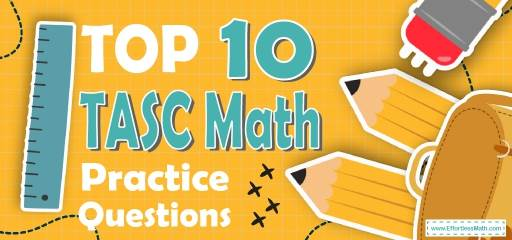# Top 10 TASC Math Practice QuestionsTaking the TASC Math test in a few weeks or even in a few days? The best way to prepare for your TASC Math test is to work through as many TASC Math practice questions as possible. Here are the top 10 TASC Math practice questions to help you review the most important TASC Math topics. These TASC Math practice questions are designed to cover mathematics concepts and topics that are found on the actual test. The questions have been fully updated to reflect the latest 2022 TASC guidelines. Answers and full explanations are provided at the end of the post.

Start your TASC Math test prep journey right now with these sample TASC Math questions.

## Sample TASC Math Practice Questions

1- When a number is subtracted from 24 and the difference is divided by that number, the result is 3. What is the value of the number?

A. $$2$$

B. $$4$$

C. $$6$$

D. $$12$$

2- An angle is equal to the one-ninth of its supplement. What is the measure of that angle?

A. $$18$$

B. $$24$$

C. $$36$$

D. $$45$$

3- John traveled 150 km in 6 hours and Alice traveled 160 km in 4 hours. What is the ratio of the average speed of John to the average speed of Alice?

A. $$3:2$$

B. $$2:3$$

C. $$5:8$$

D. $$5:6$$

4- A taxi driver earns $7 per 1-hour work. If he works 10 hours a day and in 1 hour he uses 2-liters petrol with price$1 for 1-liter. How much money does he earn in one day?

A. $90 B.$88

C. $50 D.$30

5- Find the average of the following numbers: $$12,13,7,21,22$$

A. 17

B. 16.5

C. 15

D. 11

6- The price of a sofa is decreased by $$15\%$$ to $$476$$. What was its original price?

A. $480 B.$520

C. $560 D.$600

7- Right triangle ABC has two legs of lengths 9 cm AB and 12 cm AC. What is the length of the third side BC?

A. 6 cm

B. 8 cm

C. 14 cm

D. 15 cm

8- If $$40\%$$ of a class are girls, and $$25\%$$ of girls play tennis, what percent of the class play tennis?

A. $$10\%$$

B. $$15\%$$

C. $$20\%$$

D. $$40\%$$

9- The area of a circle is less than $$36 π$$. Which of the following can be the circumference of the circle?

A. $$10 π$$

B. $$12 π$$

C. $$24 π$$

D. $$36 π$$

10- Which of the following values for x and y satisfy the following system of equations?
$$\begin{cases}x+4y=10 \\ 5x+10y=10\end{cases}$$

A. $$x=3,y=2$$

B. $$x=2,y−3$$

C. $$x=−6,y=4$$

D. $$x=4,y=−2$$

## Best TASC Math Prep Resource for 2022

1- C
Let $$x$$ be the number. Write the equation and solve for $$x.(24-x)÷x=3$$ Multiply both sides by $$x. (24-x) =3x$$, then add $$x$$ both sides. $$24=4x$$, now divide both sides by $$4. x=6$$

2- A
The sum of supplement angles is 180. Let $$x$$ be that angle. Therefore, (x+9x =180,10x=180\), divide both sides by $$10: x=18$$

3- C
The average speed of john is: $$150÷6=25$$ km, The average speed of Alice is: $$160÷4=40$$ km.Write the ratio and simplify. $$25∶40 ⇒ 5:8$$

4- C
$$7×10=70$$, Petrol use: $$10×2=20$$ liters. Petrol cost: $$20×1=20$$
Money earned: $$70-20=50$$

5- C
average $$=\frac{sum \ of \ terms}{number \ of \ terms}=\frac{12+ 13+7+21+22}{5} = \frac{75}{5} =15$$

6- C
Let x be the original price. If the price of the sofa is decreased by $$15\%$$ to $476, then: $$85\%$$ of $$x=476 ⇒ 0.85x=476 ⇒ x=476÷0.85=560$$ 7- D Use Pythagorean Theorem: $$a^2+b^2=c^2, 9^2+12^2= c^2 ⇒ 81+144=c^2⇒ 225=c^2⇒c=15$$ 8- A The percent of girls playing tennis is: $$40\%×25\% =0.40×0.25=0.10=10\%$$ 9- A The area of the circle is less than $$12 π$$. Use the formula of areas of circles. Area $$=πr^2 ⇒$$ $$36 π> πr^2⇒ 36>r^2⇒ r<6$$. The radius of the circle is less than 6. Let’s put 6 for the radius. Now, use the circumference formula: Circumference $$=2πr=2π (6)=12 π$$ Since the radius of the circle is less than 6. Then, the circumference of the circle must be less than $$12 π$$. Only choice A is less than $$12 π$$. 10- C $$\begin{cases}x+4y=10 \\ 5x+10y=10\end{cases}\rightarrow$$ Multiply the top equation by $$-5$$ then, $$\begin{cases}-5x-20y=-50 \\ 5x+10y=10\end{cases}\rightarrow$$ Add two equations $$-10y=-40→y=4$$ , plug in the value of y into the first equation $$x+4y=10→x+4(4)=10→x+16=10$$ Subtract 16 from both sides of the equation. Then: $$x+16=10→x=-6$$ Looking for the best resource to help you succeed on the TASC Math test? ## High School Equivalency Tests ## The Best Books to Ace the TASC Math Test ## More from Effortless Math for TASC Test … ### Are you looking for a complete and FREE TASC Math course to help you prepare for your TASC Math test? Check out our Ultimate TASC Math Course. ### Need Math worksheets to help you measure your exam readiness for your upcoming TASC test? Have a look at our comprehensive TASC Math Worksheets to help you practice all TASC Math concepts and prepare for the TASC Math test. ### Looking for TASC Math websites to find FREE online resources? Here is our complete list of Top 10 Websites for FREE TASC Math Preparation. ## The Perfect Prep Books for the TASC Math Test ## Have any questions about the TASC Test? Write your questions about the TASC or any other topics below and we’ll reply! ## Related to This Article ### More math articles ### What people say about "Top 10 TASC Math Practice Questions - Effortless Math: We Help Students Learn to LOVE Mathematics"? No one replied yet. X 52% OFF Limited time only! Save Over 52% SAVE$40

It was $76.99 now it is$36.99